## An electron with velocity v = 1.0 ´ 106 m/s is sent between the plates of a capacitor where the electric field is E = 500 V/m. If the distan

Question

An electron with velocity v = 1.0 ´ 106 m/s is sent between the plates of a capacitor where the electric field is E = 500 V/m. If the distance the electron travels through the field is 1.0 cm, how far is it deviated (Y) in its path when it emerges from the electric field? (me = 9.1 ´ 10 – 31 kg, e = 1.6 ´ 10 – 19 C)

in progress 0
2 months 2021-08-02T18:44:48+00:00 1 Answers 3 views 0

The deviation in path is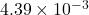Explanation:

Given:

Velocity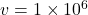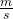Electric field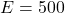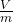Distance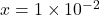m

Mass of electron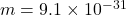kg

Charge of electron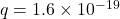C

Time taken to travel distance,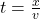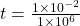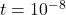sec

Acceleration is given by,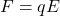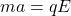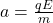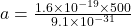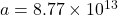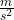For finding the distance, we use kinematics equations.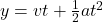Where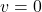because here initial velocity zero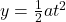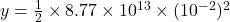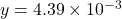m

Therefore, the deviation in path is